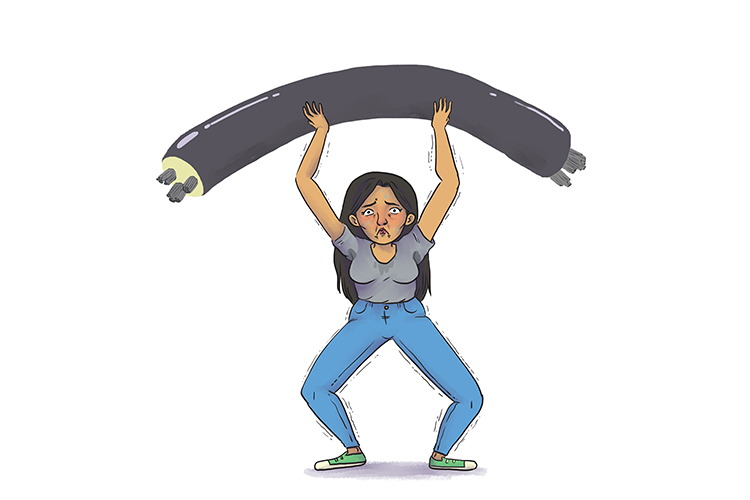# Most efficient way to transmit 1 KVA

 1000 volt and 1 Amp equal 1000W1 volt and 1000 Amp equal 1000W500 volt and 2 Amp equal 1000W5 volt and 200 Amp equal 1000W

The question becomes: which is the most efficient way to send power (voltage and current) down a wire?

Power lines have losses when heat is generated in them by electric current moving through the wires. There is a loss of power down the length of the wire.

Example 1

 Double the voltage halves the current The power loss decreases by 2^2=4

Or another way of saying this

 Increase the voltage by a factor of  2 decreases the current by a factor of  2 The power loss decreases by 2^2=4

Example 2

 Increase the voltage by a factor of  100 decreases the current by a factor of  100 The power loss decreases by 100^2=10,000

So there are massive advantages for sending high voltage over long distances in a wire.

HIGH VOLTAGE = LOWER ENERGY LOSSES

Which equals thinner cables needed.